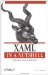# Rect3D

 Rect3D Rect3D is a structure and has no class hierarchy.

`     <Rect3D         Location="0,0,0"         Size="10 10 10"/> `

or:

`     <Rect3D         X="0"         Y="0"         Z="0"         SizeX="10"         SizeY="10"         SizeZ="10" /> `

<Rect3D .../> represents a rectangle and is most commonly used in other elements to describe rectangular regions for hit-testing or filling a region.

#### Attributes

Location (optional)

This Point3D describes the top-left corner of the rectangle. It can be described using abbreviated markup syntax or explicitly using the X, Y, and Z attributes.

SizeX (optional)

This Double value describes the size of the rectangle in the x-dimension.

SizeY (optional)

This Double value describes the size of the rectangle in the y-dimension.

SizeZ (optional)

This Double value describes the size of the rectangle in the z-dimension.

X (optional)

This Double value describes the x-coordinate of the top-left corner of the rectangle.

Y (optional)

This Double value describes the y-coordinate of the top-left corner of the rectangle.

Z (optional)

This Double value describes the z-coordinate of the top-left corner of the rectangle.XAML in a Nutshell (In a Nutshell (OReilly))
ISBN: 0596526733
EAN: 2147483647
Year: 2007
Pages: 217